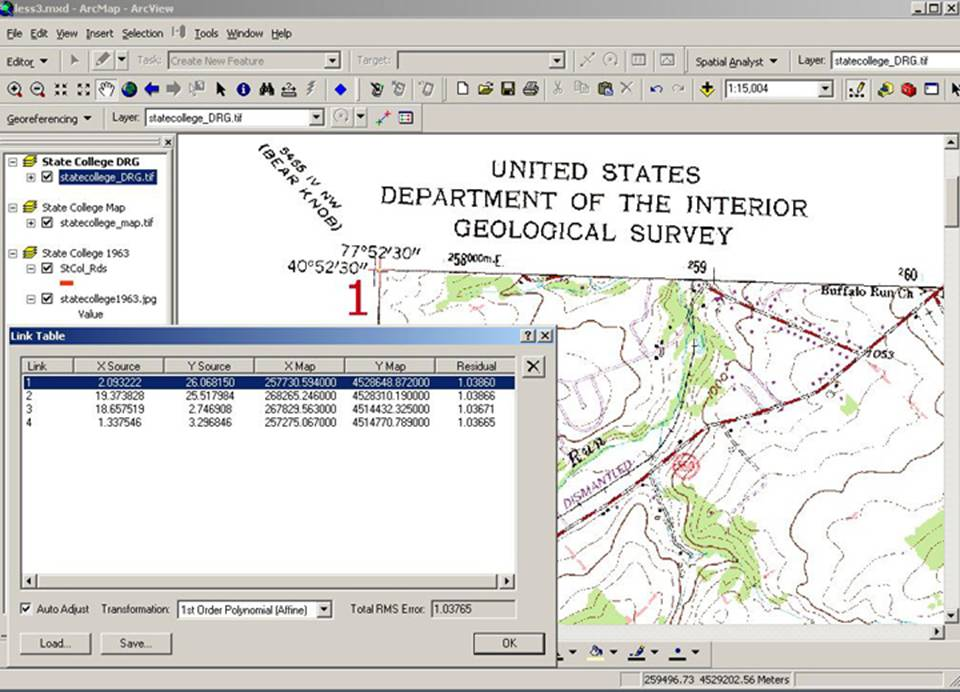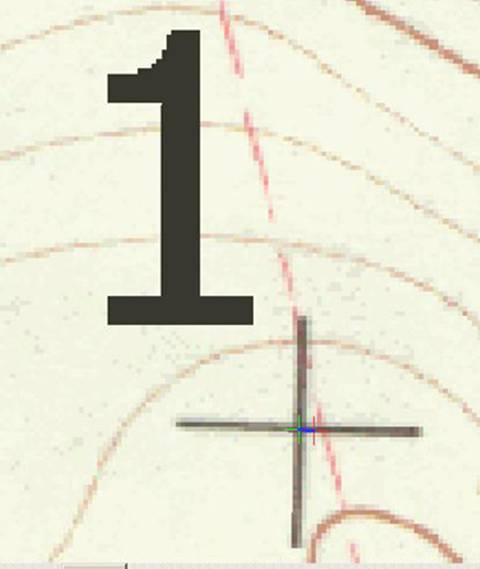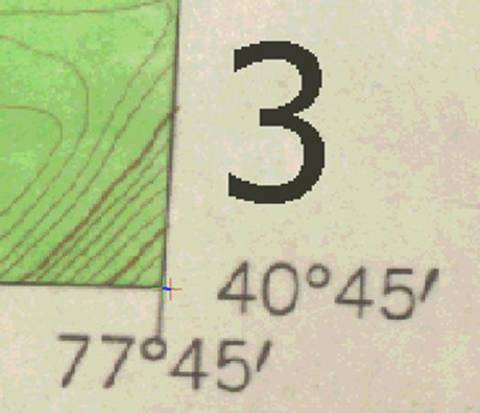Georeferencing Raster Images

by John Middendorf

Below are the steps to georeference a raster image with a layer with a known projection using ESRI ArcGIS software:

First case: DRG Georeference

First, we georeference  a digital raster graphic that has “lost” its georeferencing information.

Here we use an affine transformation using known XY control points.  Using the known UTM coordinates (Zone 18, NAD27) of the corners of the topo quad, we add four control points on the map, then edit the link table to reflect the UTM coordinates.  Once the image is georeferenced with the control points, we can permanently attach the projection information by selecting the properties of the data frame, selecting the proper projection (NAD 1927 UTM Zone 18), and then selecting “Update Georeferencing” from the Georeference toolbar.  The DRG properties will then reflect the updated projection information, with accurate cell size and extents.

To permanently create a georeferenced image, the Rectify command is then used.Above:  Georeferenced DRG graphic showing the Link Table with UTM coordinates linked to known XY points on the Tiff.

Discussion:  The total root mean square residual error is 1.04.  The cell size can be calculated with the knowledge of the scale of the map (1:24,000) and the fact that the DRG has 250 pixels per inch.  To determine the cell size, we take the resolution of 250 cells per inch, and use the scale of 1 inch equals 24,000 inches.  THUS, each cell represents 24,000/250, or 96 inches.  96 inches is 2.43 meters, the cell size in meters.  This can also be found in the statecollege_DRG.tif Raster information (after the units are changed appropriately to meters).

The RMS error in this case is less than one half of our cell size, so this is within our rule of thumb of RMS accuracy.  It has diagnostic value because the actual dimension of cell size is well known with the raster DRG.

Second case: Scanned USGS Map

In this second case, we georeference a scanned 1:24,000 scale USGS map.  A similar procedure as before is used, but instead of the four corners, we use four known points on the edges.Above: Scanned USGS topo map after georeferencing.

Here we have a large RMS error.  After we georeferenced the scanned, the cell size becomes approximately the same as the DRG image, but slightly larger, around 2.45 meters.  The cell size in this case must reflect the scanner resolution, presumably scanned at about 250 pixels per inch.  However, the scanned image has some issues; in this case, it appears that the scan resulted in a trapezoidal image, as demonstrated by the images below:Above: images showing the control points (green) and the georeferenced coordinates (red).  In cases of point 1 and 2, the georeferenced (known) coordinates are on the inside of the control points, but in cases 3 and 4 (on the bottom of the scanned image), the georeferenced coordinates are on the outside of the control points.  This indicates that the scan has some symmetry problems, with the pixels representing wider dimensions at the bottom as compared with the upper portion of the map.

Since the RMS error it nearly three times the cell size, it is not an acceptable georeference. It does have diagnostic value, assuming the scanner resolution is known.  Perhaps more control points and a second order approximation would improve the georeference.

Third case: Georeferencing an aerial photo with a vector layer with a known coordinate system and projection.

In this case, we use the generalized control point georeference methodology outlined in the beginning of this page.Above: Georeferenced aerial photo and road layer (with known projection).

In this case, since we do not have any scale or projection information of the aerial photo, even with a known cell size (0.7m, after georeferencing and rectifying), the RMS error does not have diagnostic value.  In effect what we have done in this case is rubbersheeted the aerial image to road layer with a known projection.